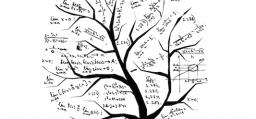# Smaller 3848

Dano had a natural number in mind. If he changed this number in a 2:5 ratio, he would get the number 24 smaller. What number did Dano think?

x =  40

### Step-by-step explanation:

x·2/5 = x - 24

3x = 120

x = 120/3 = 40

x = 40

Our simple equation calculator calculates it.Did you find an error or inaccuracy? Feel free to write us. Thank you!

Tips for related online calculators
Need help calculating sum, simplifying, or multiplying fractions? Try our fraction calculator.
Check out our ratio calculator.
Do you have a linear equation or system of equations and looking for its solution? Or do you have a quadratic equation?